# RD Sharma Solutions Class 12 Probability Exercise 31.2

RD Sharma Solutions for Class 12 Maths Chapter 31 Probability Exercise 31.2 are given here for students to prepare and score good marks in their examinations. All the fundamental concepts are outlined in detail to help students understand them easily. These solutions are developed by an expert faculty team, following the latest CBSE guidelines. Further, students can now easily access the RD Sharma Class 12 Solutions of this exercise by downloading the PDF, from the link attached below.

## Download PDF of RD Sharma Solutions for Class 12 Maths Chapter 31 Exercise 2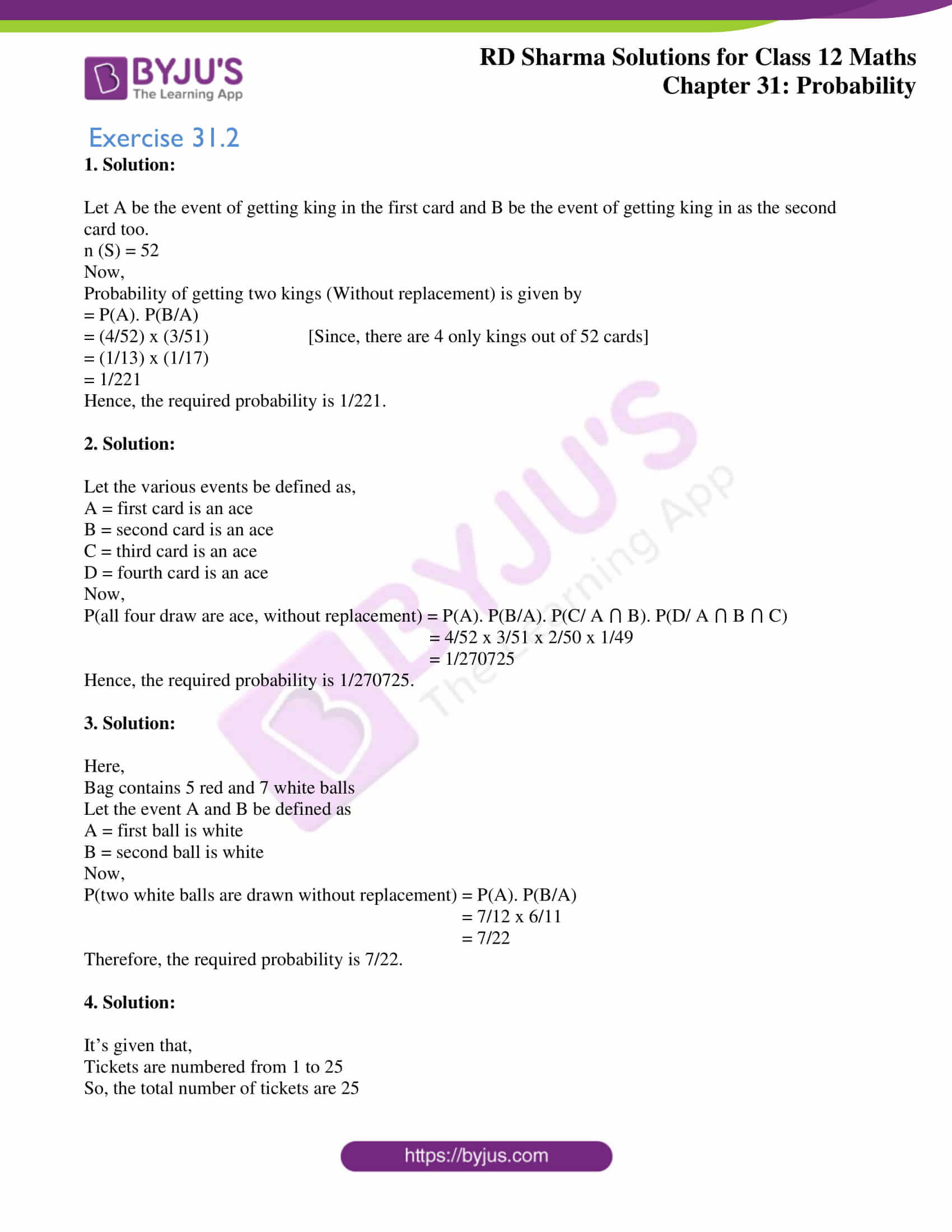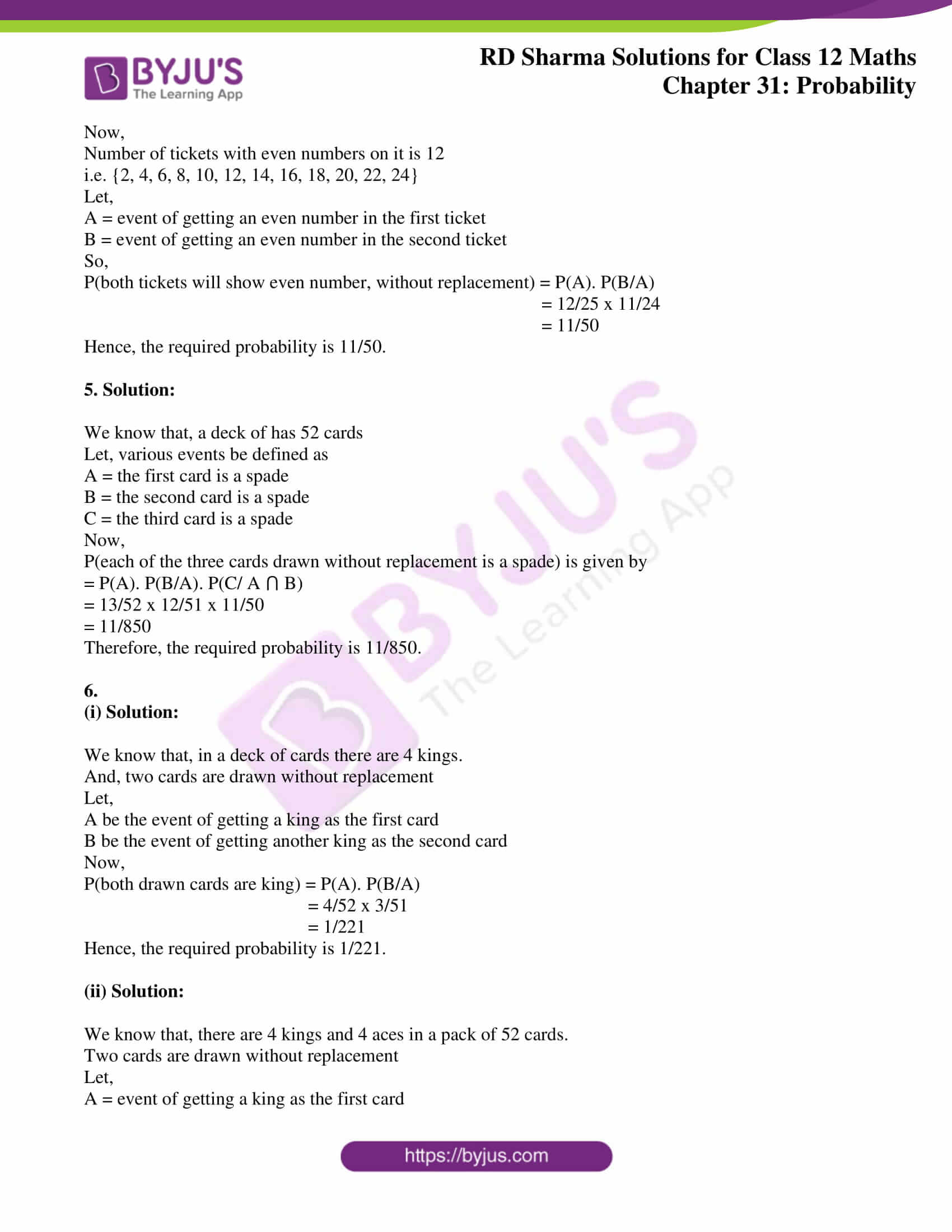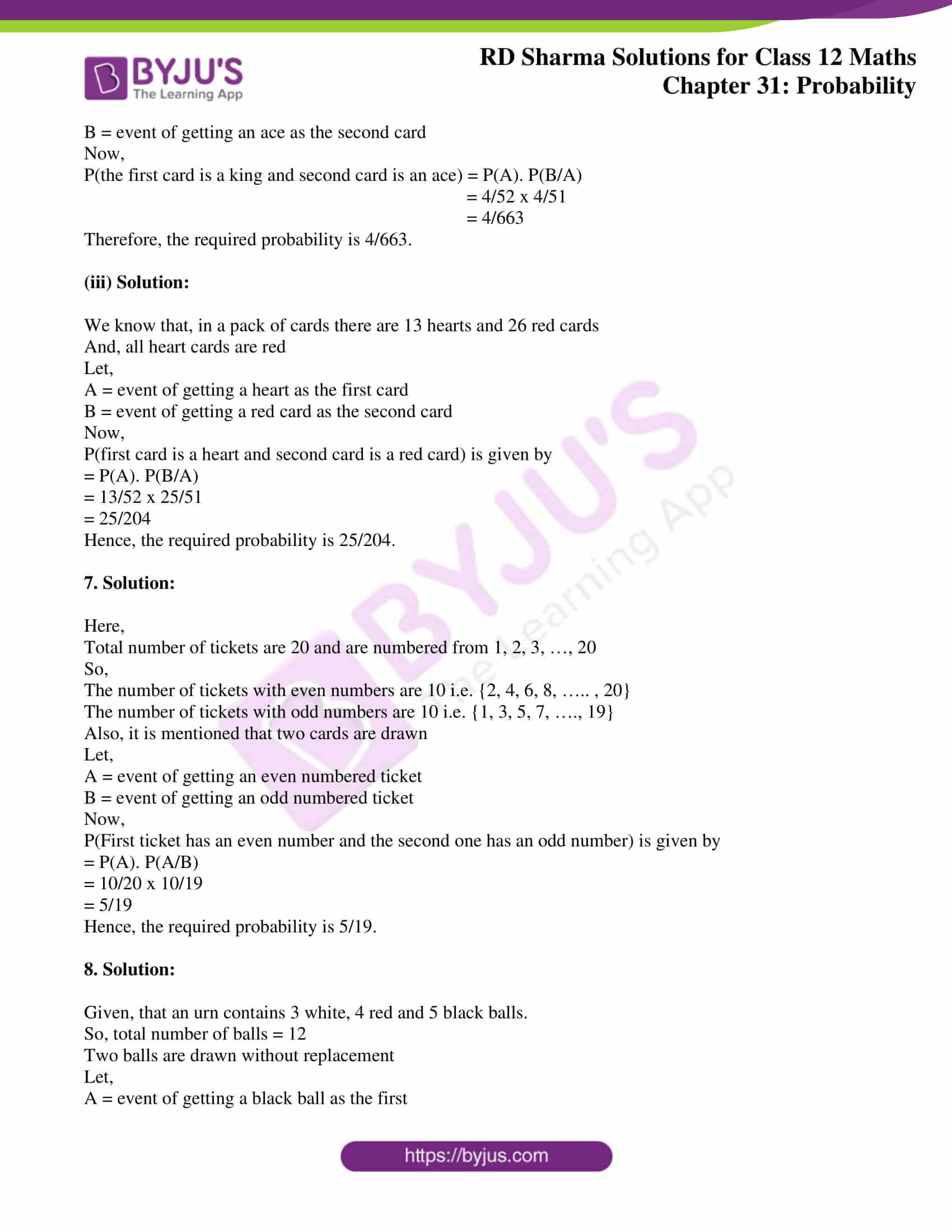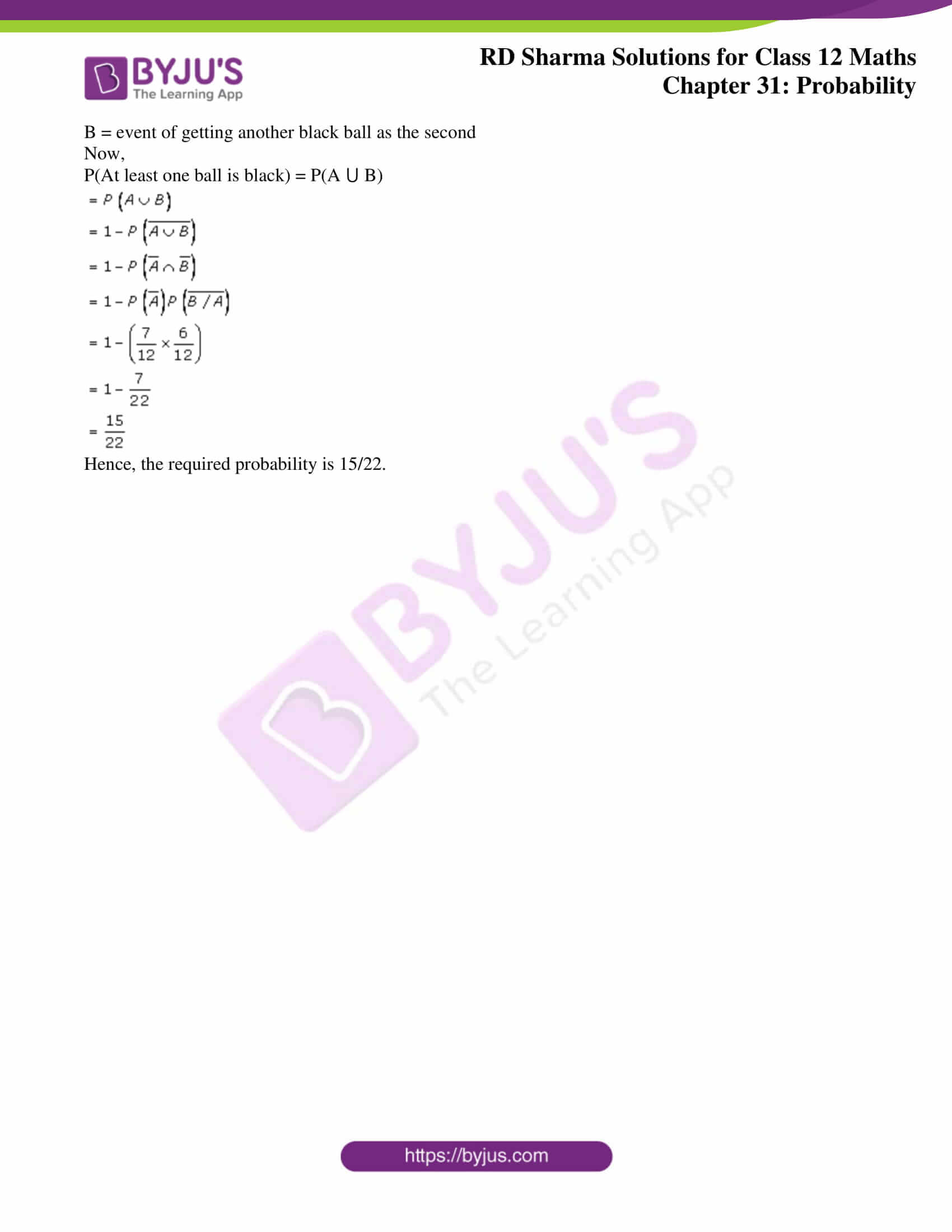### Access RD Sharma Solutions for Class 12 Maths Chapter 31 Exercise 2

Exercise 31.2

1. Solution:

Let A be the event of getting king in the first card and B be the event of getting king in as the second card too.

n (S) = 52

Now,

Probability of getting two kings (Without replacement) is given by

= P(A). P(B/A)

= (4/52) x (3/51) [Since, there are 4 only kings out of 52 cards]

= (1/13) x (1/17)

= 1/221

Hence, the required probability is 1/221.

2. Solution:

Let the various events be defined as,

A = first card is an ace

B = second card is an ace

C = third card is an ace

D = fourth card is an ace

Now,

P(all four draw are ace, without replacement) = P(A). P(B/A). P(C/ A ⋂ B). P(D/ A ⋂ B ⋂ C)

= 4/52 x 3/51 x 2/50 x 1/49

= 1/270725

Hence, the required probability is 1/270725.

3. Solution:

Here,

Bag contains 5 red and 7 white balls

Let the event A and B be defined as

A = first ball is white

B = second ball is white

Now,

P(two white balls are drawn without replacement) = P(A). P(B/A)

= 7/12 x 6/11

= 7/22

Therefore, the required probability is 7/22.

4. Solution:

It’s given that,

Tickets are numbered from 1 to 25

So, the total number of tickets are 25

Now,

Number of tickets with even numbers on it is 12

i.e. {2, 4, 6, 8, 10, 12, 14, 16, 18, 20, 22, 24}

Let,

A = event of getting an even number in the first ticket

B = event of getting an even number in the second ticket

So,

P(both tickets will show even number, without replacement) = P(A). P(B/A)

= 12/25 x 11/24

= 11/50

Hence, the required probability is 11/50.

5. Solution:

We know that, a deck of has 52 cards

Let, various events be defined as

A = the first card is a spade

B = the second card is a spade

C = the third card is a spade

Now,

P(each of the three cards drawn without replacement is a spade) is given by

= P(A). P(B/A). P(C/ A ⋂ B)

= 13/52 x 12/51 x 11/50

= 11/850

Therefore, the required probability is 11/850.

6.

(i) Solution:

We know that, in a deck of cards there are 4 kings.

And, two cards are drawn without replacement

Let,

A be the event of getting a king as the first card

B be the event of getting another king as the second card

Now,

P(both drawn cards are king) = P(A). P(B/A)

= 4/52 x 3/51

= 1/221

Hence, the required probability is 1/221.

(ii) Solution:

We know that, there are 4 kings and 4 aces in a pack of 52 cards.

Two cards are drawn without replacement

Let,

A = event of getting a king as the first card

B = event of getting an ace as the second card

Now,

P(the first card is a king and second card is an ace) = P(A). P(B/A)

= 4/52 x 4/51

= 4/663

Therefore, the required probability is 4/663.

(iii) Solution:

We know that, in a pack of cards there are 13 hearts and 26 red cards

And, all heart cards are red

Let,

A = event of getting a heart as the first card

B = event of getting a red card as the second card

Now,

P(first card is a heart and second card is a red card) is given by

= P(A). P(B/A)

= 13/52 x 25/51

= 25/204

Hence, the required probability is 25/204.

7. Solution:

Here,

Total number of tickets are 20 and are numbered from 1, 2, 3, …, 20

So,

The number of tickets with even numbers are 10 i.e. {2, 4, 6, 8, ….. , 20}

The number of tickets with odd numbers are 10 i.e. {1, 3, 5, 7, …., 19}

Also, it is mentioned that two cards are drawn

Let,

A = event of getting an even numbered ticket

B = event of getting an odd numbered ticket

Now,

P(First ticket has an even number and the second one has an odd number) is given by

= P(A). P(A/B)

= 10/20 x 10/19

= 5/19

Hence, the required probability is 5/19.

8. Solution:

Given, that an urn contains 3 white, 4 red and 5 black balls.

So, total number of balls = 12

Two balls are drawn without replacement

Let,

A = event of getting a black ball as the first

B = event of getting another black ball as the second

Now,

P(At least one ball is black) = P(A ⋃ B)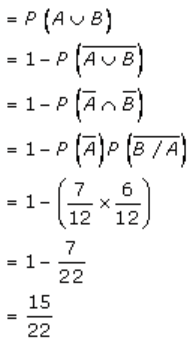Hence, the required probability is 15/22.